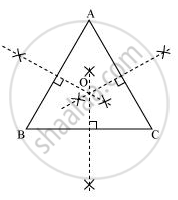# ABC is a triangle. Locate a point in the interior of ΔABC which is equidistant from all the vertices of ΔABC. - Mathematics

ABC is a triangle. Locate a point in the interior of ΔABC which is equidistant from all the vertices of ΔABC.

#### Solution

Circumcentre of a triangle is always equidistant from all the vertices of that triangle. Circumcentre is the point where perpendicular bisectors of all the sides of the triangle meet together.In ΔABC, we can find the circumcentre by drawing the perpendicular bisectors of sides AB, BC, and CA of this triangle. O is the point where these bisectors are meeting together. Therefore, O is the point which is equidistant from all the vertices of ΔABC.

Concept: Inequalities in a Triangle
Is there an error in this question or solution?
Chapter 7: Triangles - Exercise 7.5 [Page 133]

#### APPEARS IN

NCERT Class 9 Maths
Chapter 7 Triangles
Exercise 7.5 | Q 1 | Page 133

Share